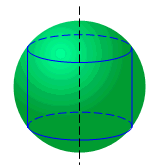Ch 4. The Mean Value Theorem Multimedia Engineering Math Maximum & Minimum Rolle'sTheorem Mean ValueTheorem MonotonicFunctions First DerivativeTest Concavity &Inflection SecondDerivative Test
 Chapter 1. Limits 2. Derivatives I 3. Derivatives II 4. Mean Value 5. Curve Sketching 6. Integrals 7. Inverse Functions 8. Integration Tech. 9. Integrate App. 10. Parametric Eqs. 11. Polar Coord. 12. Series Appendix Basic Math Units Search eBooks Dynamics Fluids Math Mechanics Statics Thermodynamics Author(s): Hengzhong Wen Chean Chin Ngo Meirong Huang Kurt Gramoll ©Kurt GramollMATHEMATICS - CASE STUDY IntroductionInscribe Cylinder in a Sphere While repairing an old washing machine, Wisdom finds a cylinder balance weight needs to be replaced. In order to save money, Wisdom decides to make this part by cutting a steel sphere. He wants the cylinder balance weight heaviest. What is known: The radius of the sphere is 0.05 m. The mass density of this sphere is 7,900 kg/m3. The cylinder is inscribed in the sphere. The weight of the cylinder, w, is determined by its mass density, ρ, and volume, v. Questions What can the maximum weight the cylinder be? Approach Find the volume that can be inscribed in the sphere. Using the second derivative test to verify the volume is maximum.